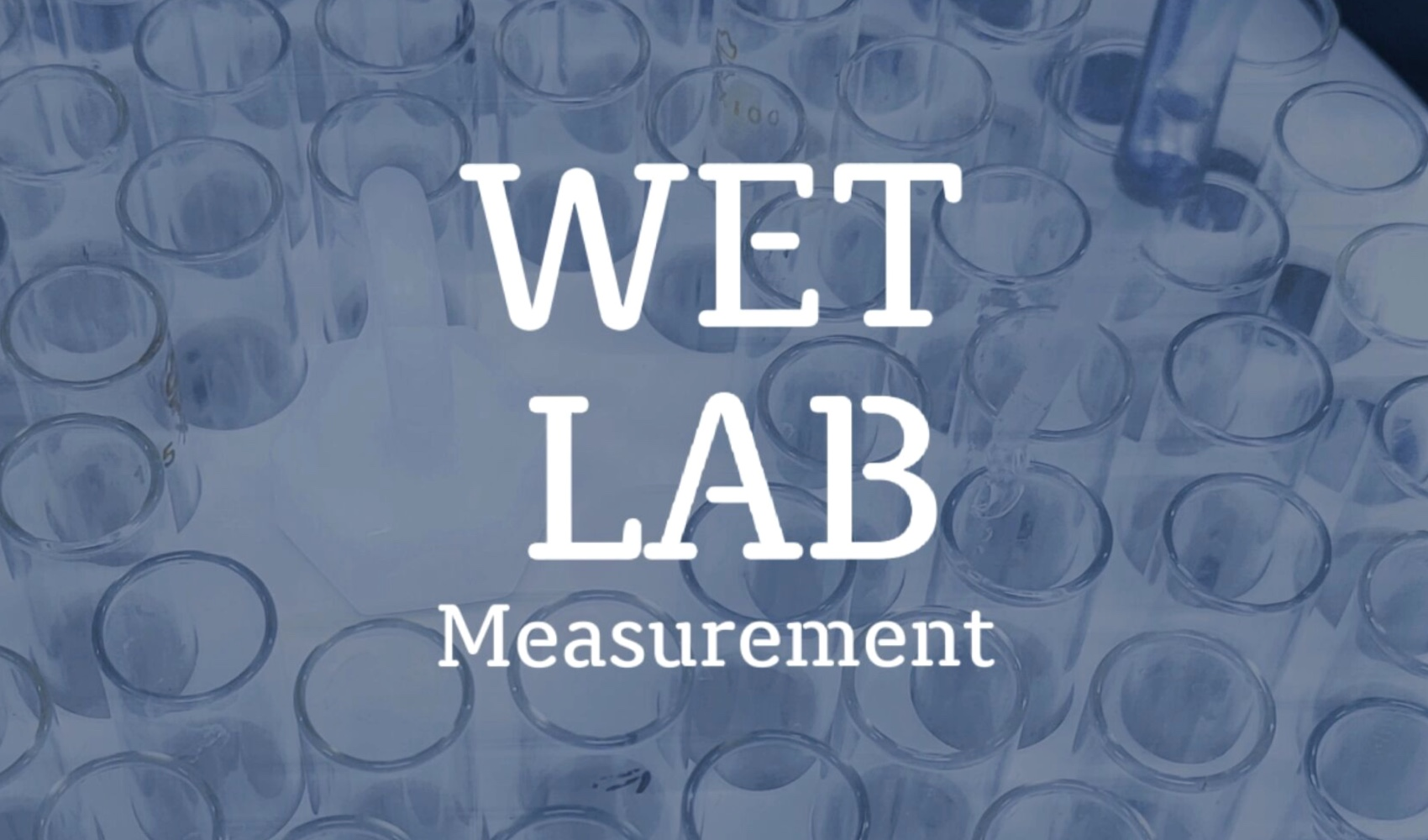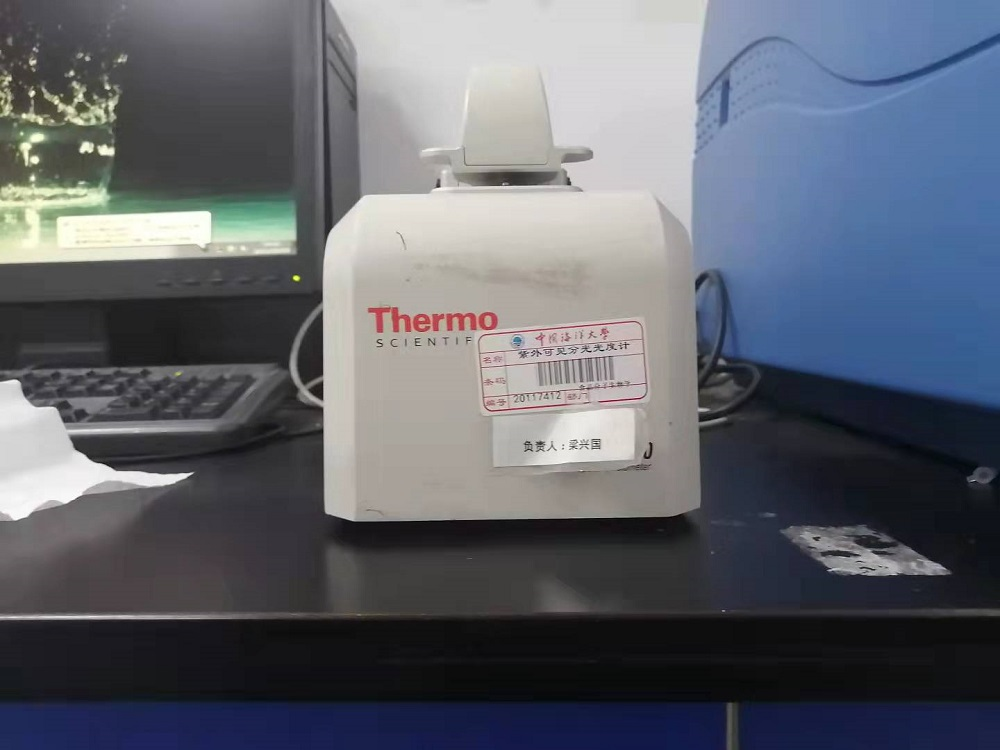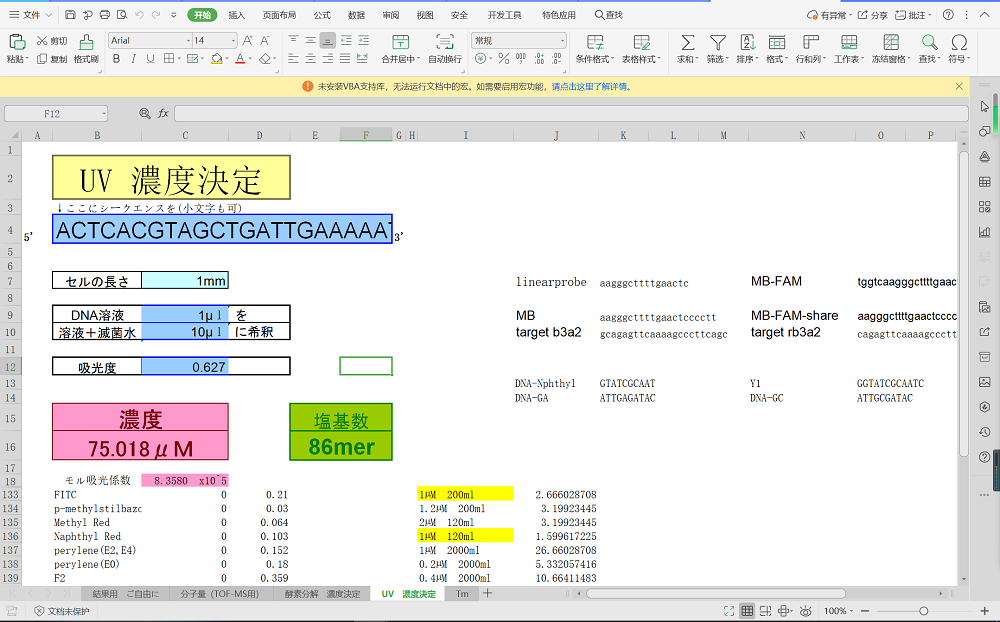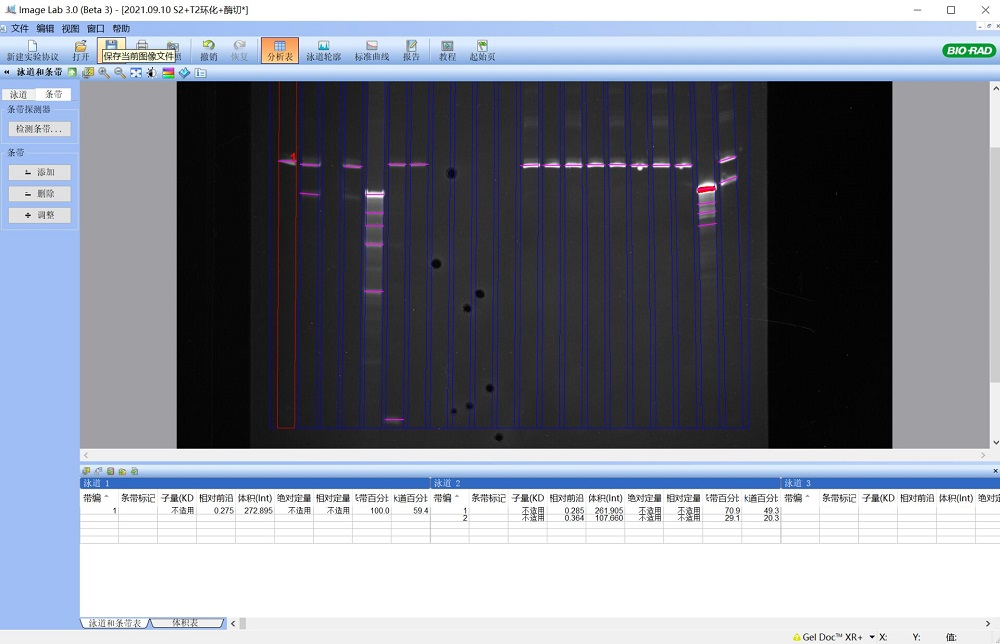# Team:OUC-R/Measurement# Measurement

## Measure the absorbance and analyze the sample concentration

Sample concentration was measured with absorbance analysis

After we get the samples ordered from the company, we need to measure the concentration of the samples.The method we use is to measure the absorbance of the sample and calculate the sample concentration according to the absorbance.We used nanodrope to measure the uv absorbance of our samples.We chose to measure the absorbance of DNA samples under the condition of OD=260nm, because the nucleic acid has the maximum uv absorption at 260nm wavelength.DNA samples were diluted and absorbance was measured using nanodrope three times for each sample.We averaged the data and used a specific algorithm to calculate the concentration of the DNA sample.## The yield was analyzed by electrophoretic diagram

After cyclization and enzymatic digestion, we need to measure the cyclization rate of the sample.In addition, the yield needs to be calculated after the topological isomers are prepared.Here we measure our yield by analyzing the results of electrophoresis.We used gel imager to obtain the electrophoretic images of our experiment, and analyzed the obtained electrophoretic images by Imagelab.According to the data obtained by the software, we calculated our yield and further obtained the concentration of the product for the convenience of subsequent experimental operations.